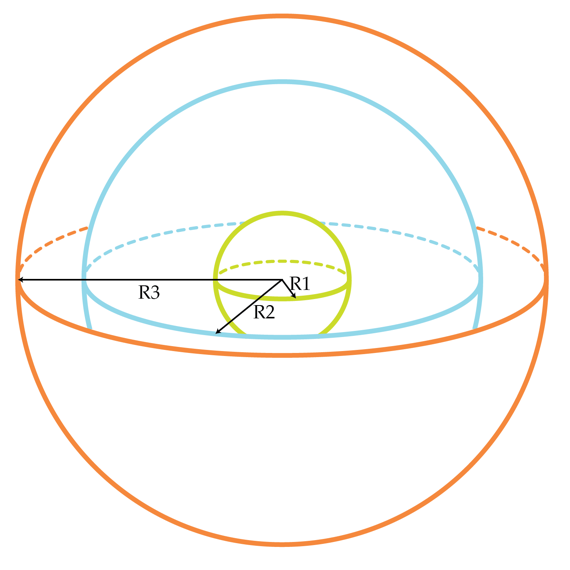# Metallic Spheres

Consider a system of three concentric metallic spheres with radii $R_{1}= 10 cm, R_{2}=30 cm$ and $R_{3}=40 cm$. The inner ($R_{1}$) and the outer ($R_{3}$) spheres are grounded (they are connected to a large conductor at zero potential ). The relation between the charge Q of the middle sphere and its potential V is linear $Q= C_{self} V.$ The coefficient $C_{self}$ is called self-capacitance. Determine $C_{self}$ in Farads for the middle sphere.Details and assumptions

$k=\frac{1}{4\pi \epsilon_{0}}= 9\times 10^{9} m/F$

×